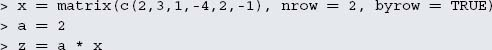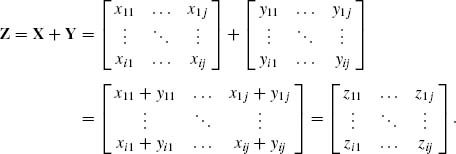Appendix B

Matrices: An introduction to matrix algebra

B.1 Multiplication by a constant

Let us define matrices X and Y with i rows and j columns (i × j):When multiplying a matrix by a constant each matrix element has to be multiplied:Example
A matrixmultiplied by a constant a = 2, gives the matrix Z:In order to load a matrix X and carry out multiplication by a constant, the following commands should be typed into R (Appendix D):The output is as follows:Adding (or subtracting) matrices can be done only for matrices of the same order. When X and Y are i × j matrices, the corresponding xij and yij elements are summed (or subtracted) to give a resulting matrix Z of the same size i × j:Example
In R:
The output is as follows:

The properties of ...

Get Statistical Analysis in Forensic Science: Evidential Values of Multivariate Physicochemical Data now with the O’Reilly learning platform.

O’Reilly members experience books, live events, courses curated by job role, and more from O’Reilly and nearly 200 top publishers.